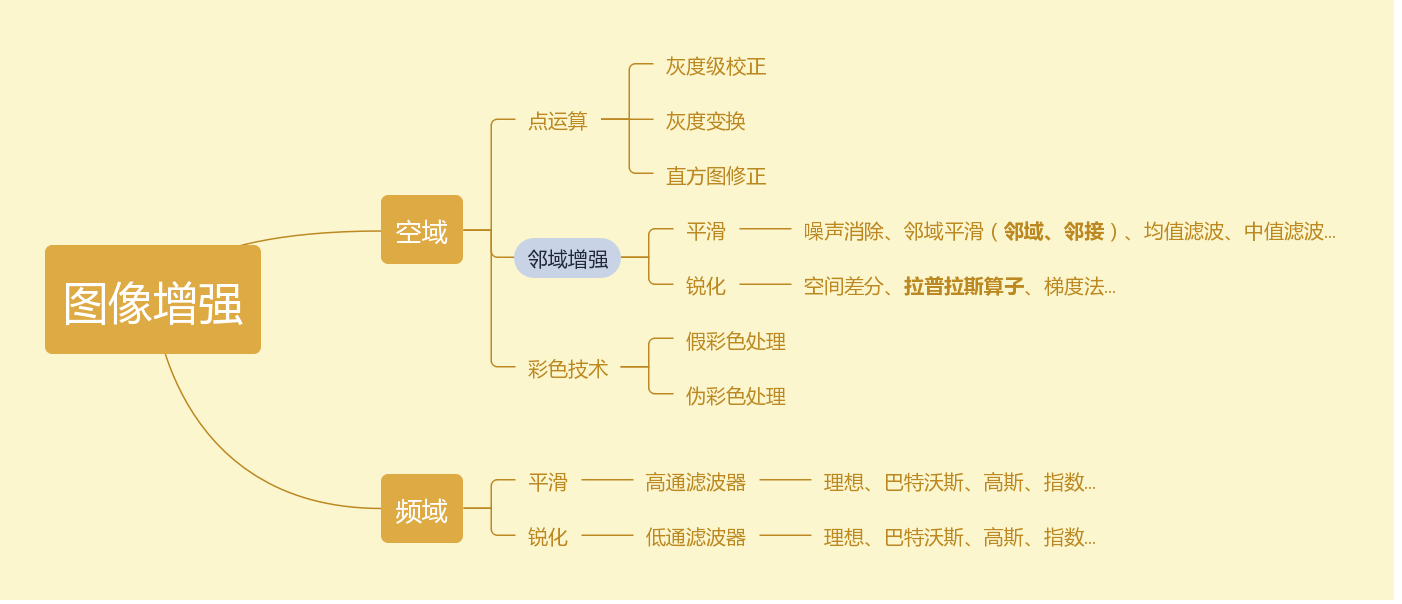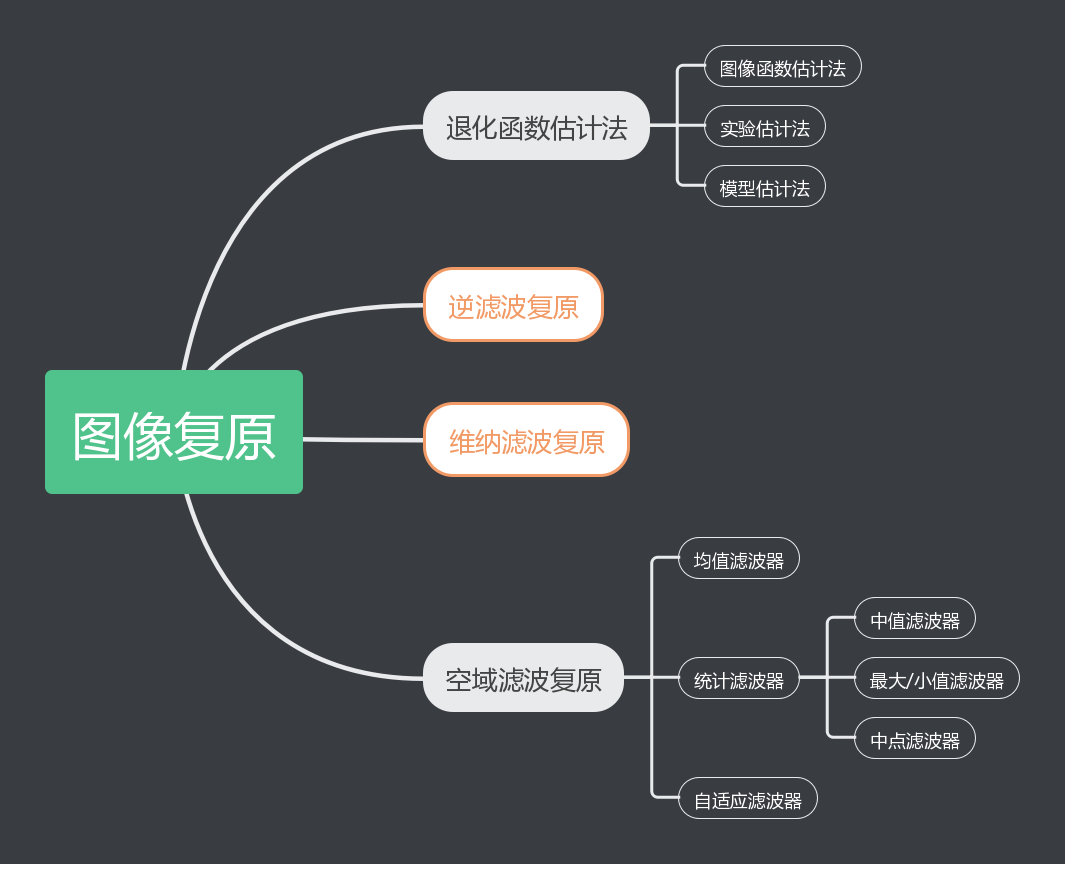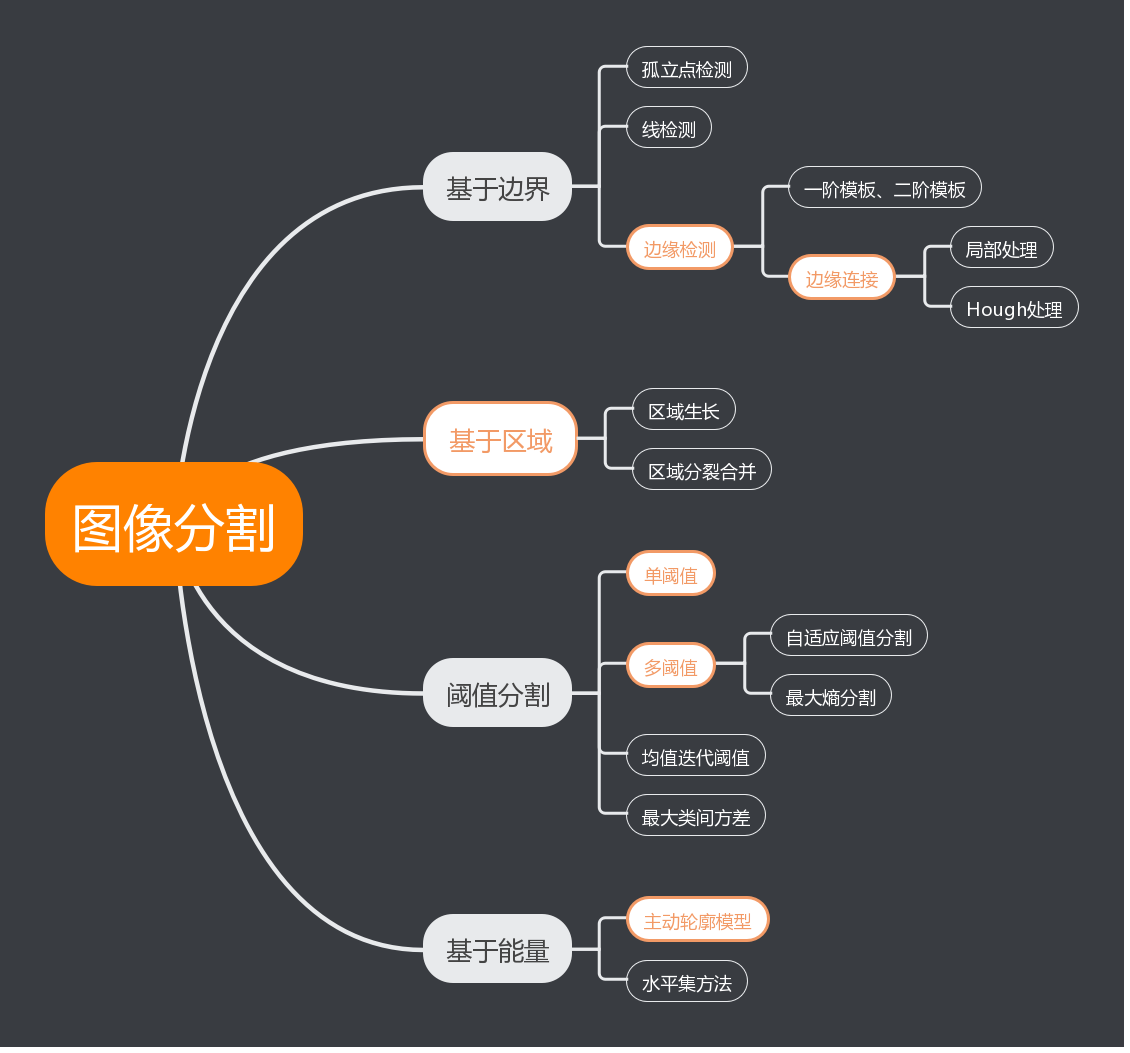2017-07-25 15:51:23 qq_35954333 阅读数 1944
• ###### 微信小程序界面设计-小程序中的WXSS(css)选择器

讲解微信小程序中如何使用css选择器，哪些选择器支持哪些选择器不支持，讲解选择器分组,属性选择器,后代选择器,子元素和相邻兄弟选择器,伪类选择器,伪元素选择器,微信小程序中样式css选择器等

1177 人正在学习 去看看 黄菊华

1.种子点获取。

2.种子点入栈，考虑种子点周围八领域灰度值，若邻域像素满足生长条件，则该点入栈。

3.获取栈顶元素，考虑其邻域像素，若满足则入栈，不满则舍弃，重复该过程直至栈为空。

4.获取下一个种子点，重复2,3直到所有种子点已全部完成生长，程序结束。

```class ImageGrow	//区域生长类
{
public:
cv::Mat Image;	//原图像
cv::Mat Image_Grow;	//标记图像
std::vector<cv::Point>	seed;	//待生长种子点
std::vector<cv::Point>	region_grow_point;	//生长后像素点集

public:

void Region_Grow(int);		//生长算法
void Show_Image();	//显示生长后图像

};

void ImageGrow::Region_Grow(int thre)
{
cv::Mat flagmat=cv::Mat::zeros(Image.size(),CV_8U);	//用于标记区域生长情况
std::stack<cv::Point>	pointstack;		//用于存放像素点的栈
std::vector<cv::Point2i>	direction(8);	//8邻域编码，用于遍历生长点周围像素
direction=cv::Point2i(-1,-1);
direction=cv::Point2i(-1,0);
direction=cv::Point2i(-1,1);
direction=cv::Point2i(0,-1);
direction=cv::Point2i(0,1);
direction=cv::Point2i(1,-1);
direction=cv::Point2i(1,0);
direction=cv::Point2i(1,1);

cv::Point2i src,test_src;
int src_value;

for(int i=0;i<seed.size();++i)	//种子点入栈
{
src=seed[i];
pointstack.push(src);

flagmat.at<uchar>(src.y,src.x)=255;

src_value=Image.at<uchar>(src.y,src.x);

while(!pointstack.empty())	//栈非空则一直执行生长算法
{
src=pointstack.top();	//提取栈顶像素点

region_grow_point.push_back(src);

pointstack.pop();

for(int j=0;j<direction.size();++j)	//8邻域判别
{
test_src.x=src.x+direction[j].x;
test_src.y=src.y+direction[j].y;

if(test_src.x<0 || test_src.y<0 || test_src.x>(Image.cols-1) || test_src.y>(Image.rows-1))
continue;
//检查像素点是否越界
if(!flagmat.at<uchar>(test_src.y,test_src.x))//若该像素点之前没有被标记过则对该像素点进行判别
{
int cur_value=Image.at<uchar>(test_src.y,test_src.x);
if(abs(src_value-cur_value)<thre)	//符合生长条件，像素点入栈
{

flagmat.at<uchar>(test_src.y,test_src.x)=255;
pointstack.push(test_src);
}
}
}
}

}
Image_Grow=flagmat.clone();

}
void ImageGrow::Show_Image()		//显示图像
{
cv::Mat	Image_Color(Image.size(),CV_8UC3,cv::Scalar(0,0,0));//创建三通道图像
std::vector<cv::Mat>	channels;
cv::split(Image_Color,channels);
channels=Image.clone();
channels=Image.clone();
channels=Image.clone();
cv::RNG	rng;
int a=rng.uniform(0,255),b=rng.uniform(0,255),c=rng.uniform(0,255);	//获取随机数，用于显示生长区域

for(int i=0;i<region_grow_point.size();++i)		//对符合条件的生长点赋值
{

channels.at<uchar>(region_grow_point[i].y,region_grow_point[i].x)=a;
channels.at<uchar>(region_grow_point[i].y,region_grow_point[i].x)=b;
channels.at<uchar>(region_grow_point[i].y,region_grow_point[i].x)=c;

}
cv::merge(channels,Image_Color);

cv::namedWindow("Image2",CV_WINDOW_NORMAL);
cv::imshow("Image2",Image_Color);

cv::imwrite("the grow Image.jpg",Image_Color);
}
int main()
{
ImageGrow Test;		//创建区域生长类对象

Test.seed.push_back(cv::Point2i(600,500));//设置生长点，这边采用手动标记方式

Test.Region_Grow(100);						//区域生长，设置生长阈值，可根据实际情况设定

cv::namedWindow("Image1",CV_WINDOW_NORMAL);
cv::imshow("Image1",Test.Image);

Test.Show_Image();							//显示图像
cv::imwrite("the origion Image.jpg",Test.Image);

cv::waitKey(-1);

return 0;
}```2019-09-20 16:52:49 OpenSceneGraph 阅读数 477
• ###### 微信小程序界面设计-小程序中的WXSS(css)选择器

讲解微信小程序中如何使用css选择器，哪些选择器支持哪些选择器不支持，讲解选择器分组,属性选择器,后代选择器,子元素和相邻兄弟选择器,伪类选择器,伪元素选择器,微信小程序中样式css选择器等

1177 人正在学习 去看看 黄菊华

1.图像增强：对于噪声图像、模糊图像等对图像信息增强以突出有用信息。• 高通平滑、低通锐化；平滑模糊、锐化突出图像细节
• 滤波器还有带通、带阻等形式
• 根据噪声（椒盐噪声、高斯噪声....）的不同，选用不同的滤波
• 邻域有4-邻域、对角邻域、8-邻域，相对应的有邻接，即空间上相邻、像素灰度相似
• 图像边缘处理：忽略不处理、补充、循环使用
• 目前尚未图像处理大多基于灰度图像
``````% %低通滤波器，filtertype-滤波器的种类，n-滤波器的阶数，image-待滤波的原图像
% function H=lpfilter(filtertype,D0,n,image)
% [DIM1,DIM2,K]=size(im);%确定原始图像大小
% if K==3
%     im1=rgb2hsv(im);
%     im2=double(im1(:,:,3));
% else
%     im2=double(im);
% end
% u=0:(DIM1-1);
% v=0:(DIM2-1);
% idx=find(u>DIM1/2);
% u(idx)=u(idx)-DIM1;
% idy=find(v>DIM2/2);
% v(idy)=v(idy)-DIM2;
% [V,U]=meshgrid(v,u);
% D=sqrt(U.^2+V.^2);%计算坐标到频域中心的距离
%
% switch filtertype
%     case 'ideal'%理想
%         H=double(D<=D0);
%     case 'butterworth'%巴特沃斯
%         H=1./(1+(D./D0).^(2*n));
%     case 'guassian'%高斯
%         H=exp(-(D.^2)./(2*(D0^2)));
%     case 'exponent'%指数低通
%         H=exp(-D./(2*D0)./(2*D0));
%     case 'trapezoid'%梯形低通
%         if D<=D0
%             H=1.0;
%         else if D>100
%                 H=0.0;
%             else
%                 H=(D-100)./(D0-100);
%             end
%         end
%     otherwise
%         error('Unkown filter type.');
% end
% F=(fft2(im2));
% G=H.*F;
% g=real((ifft2(G)));
% figure;
% subplot(1,2,1);
% imshow(im2,[]);
% title('Original Image');%原始图像灰度部分
% subplot(1,2,2);
% imshow(g,[]);
% title('Lowpass Filtered Image');%低通效果
%H=lpfilter('ideal',14,1,'cat.jpg');

%低通滤波器，filtertype-滤波器的种类，n-滤波器的阶数，image-待滤波的原图像
function H=lpfilter(filtertype,D0,n,image)
[DIM1,DIM2,K]=size(im);%确定原始图像大小
if K==3
im1=rgb2hsv(im);
im2=double(im1(:,:,3));
else
im2=double(im);
end
u=0:(DIM1-1);
v=0:(DIM2-1);
idx=find(u>DIM1/2);
u(idx)=u(idx)-DIM1;
idy=find(v>DIM2/2);
v(idy)=v(idy)-DIM2;
[V,U]=meshgrid(v,u);
D=sqrt(U.^2+V.^2);%计算坐标到频域中心的距离

switch filtertype
case 'ideal'%理想
Hlp=double(D<=D0);
case 'butterworth'%巴特沃斯
if nargin==4
n=1;
end
Hlp=1./(1+(D./D0).^(2*n));
case 'guassian'%高斯
Hlp=exp(-(D.^2)./(2*(D0^2)));
otherwise
error('Unkown filter type.');
end
H=1-Hlp;
F=(fft2(im2));
G=H.*F;
g=real((ifft2(G)));
figure;
subplot(1,2,1);
imshow(im2,[]);
title('Original Image');%原始图像灰度部分
subplot(1,2,2);
imshow(g,[]);
title('Lowpass Filtered Image');%低通效果``````

2.图像复原：改善图像质量（模糊、失真、噪声）• 逆滤波法无噪声下可精确复原
• 维纳滤波法需要较多先验知识，实现难度大
• 最大熵恢复法是非线性算法，耗时
• 卡尔曼滤波法要根据前一个估计值和最近一个观测数据，计算量大
• 传播波方程恢复法利用物理知识复原，但对运动方向敏感
• 逆滤波复原形式简单，适合极高信噪比条件，但计算量大
• 维纳滤波对不同区域误差不敏感，不能处理非平稳信号和噪声
• 算术平均滤波器可消噪，平滑但模糊
• 几何平均滤波器平滑效果更好，丢失细节少
• 谐波均值滤波器处理高斯、盐噪声效果好，不适应椒噪声
• 谐逆波滤波器不同情况下适用椒、盐噪声
• 中值滤波器适用椒盐噪声
• 最大/小值滤波器适用椒/盐噪声
• 中点滤波器适用高斯、均匀噪声
``````% %逆滤波复原
% L=10;
% T=10;
% PSF=fspecial('motion',L,T);
% Blurred=imfilter(I,PSF,'circular','conv');
% subplot(2,2,1);imshow(I);
% subplot(2,2,2);imshow(Blurred);
% R=deconvwnr(Blurred,PSF);
% subplot(2,2,3);imshow(R);``````

3.图像分割注重方法的实现：阈值分割、基于区域、基于能量及有些方法的改进。

``````%Hough变换
clc;
clear all;
I=rgb2gray(I);
subplot(1,2,1);imshow(I);
rotI=imrotate(I,33,'crop');%imrotate(A,angle),将图像A围绕其中心点进行angle角度的逆时针旋转
BW=edge(rotI,'canny');%采用灰度或一个二值化图像I作为它的输入，并返回一个与I相同大小的二值化图像BW，在函数检测到边缘的地方为1，其他地方为0。
[H,T,R]=hough(BW);
subplot(1,2,2);imshow(H,[],'XData',T,'YData',R,'InitialMagnification','fit');``````
``````% %均值迭代分割
% [w,h]=size(I);
% %1--初始化
% avg=0.0;%图像的平均值
% for y=1:h
% for x=1:w
%     avg=avg+double(I(x,y));
% end
% end
% Thresh=avg./w./h;%选择一个初始化的阈值T（通常取灰度值的平均值）
% %2--将图像像素分为两类：G1：灰度值>T，G2：灰度值<T
% %计算G1中所有像素的均值u1，以及G2中所有的均值u2
% curThd=Thresh;
% preThd=curThd;
% subthd=2;
% while (subthd>1.0)
%     preThd=curThd;
%     u1=0,u2=0;
%     num_u1=0,num_u2=0;
%     for y=1:h
%     for x=1:w
%         if double(I(x,y))<preThd
%             u1=u1+double(I(x,y));
%             num_u1=num_u1+1;
%         else
%             u2=u2+double(I(x,y));
%             num_u2=num_u2+1;
%         end
%     end
%     end
%     curThd=(u1./num_u1+u2./num_u2)./2;
%     subthd=abs(preThd-curThd);
% end
% for y=1:h
%     for x=1:w
%         if double(I(x,y))<curThd
%             I(x,y)=0;
%         else
%             I(x,y)=255;
%         end
%     end
% end
% R=I;
% figure,imshow(R);

%最大类间方差分割法
level=graythresh(I);%：使用最大类间方差法找到图片的一个合适的阈值
R=im2bw(I,level);%将灰度图像转换为二值图像时，需要设定一个阈值，这个函数可以帮助我们获得一个合适的阈值
figure,imshow(R);``````

2015-07-31 15:55:36 Ben_Ben_Niao 阅读数 884
• ###### 微信小程序界面设计-小程序中的WXSS(css)选择器

讲解微信小程序中如何使用css选择器，哪些选择器支持哪些选择器不支持，讲解选择器分组,属性选择器,后代选择器,子元素和相邻兄弟选择器,伪类选择器,伪元素选择器,微信小程序中样式css选择器等

1177 人正在学习 去看看 黄菊华

1. 基于边缘检测：检测局部特性的不连续性，链接成边界，然后分割出不同的区域。包括边缘家的测图像分割、阈值选取的图像分割。
2. 区域生长：检测相邻区域的相似性，相似则是同一个区域，然后合并，不想似，则是不同的区域。包括：区域生长，分裂-合并分割方法。
3. 这两种方法可以结合一起使用。

1. 边缘检测：梯度，canny，sobel算子（前面文章已经分析过了）
2. 局部链接算法：领域内两个边缘点，当边缘梯度方向相似度超过阈值则链接边界；1. 边缘点+梯度，则可以求出具体的直线方程y=ax+b,然后将方程转换到极坐标，统计极坐标下（seta，p）的个数。后续过程如图：
2. 求出直线后，怎么求线段呢？（个人认为）求出直线后，直线的端点（x，y）在a_o_b平面肯定对应特殊的（seta,p）曲线，找出这个，然后反算到图像坐标。2005-03-14 19:44:00 jasonme 阅读数 3305
• ###### 微信小程序界面设计-小程序中的WXSS(css)选择器

讲解微信小程序中如何使用css选择器，哪些选择器支持哪些选择器不支持，讲解选择器分组,属性选择器,后代选择器,子元素和相邻兄弟选择器,伪类选择器,伪元素选择器,微信小程序中样式css选择器等

1177 人正在学习 去看看 黄菊华

3.4 图像模型

3.4.1 预测图像编码

B C
A X

3.4.2 变换编码
3.4.2.1 总述

1. 在转换域中的数据是去相关性的(转换域中的数据的大多数能量都会聚集到很小的值中)
2. 转换应该是可逆的.
3. 转换的计算过程应该是易于处理的，

3.4.2.2

X是一个采样的矩阵，Y是一个系统的矩阵，A是一个N*N的变换矩阵。A的元素是：

A(i,j) = C(i) * cos [(2j+1)*i*PI]/2N
C(i)=   N^(-1/2) i=0
(2/N)^(-1/2) i>0

3.4.2.3 小波

3.4.3 量化

3.4.3.1 标量量化

FQ = round (X/QP)
Y=FQ * QP

3.4.3.2 向量量化

1. 把原始图像分区(比如M × N像素块)
2. 从编码表中选择一个与当前区域最相近的向量
3. 把选定的向量的序号传给解码器
4. 在解码端，用选定的向量来重建一个原始图像的拷贝。

3.4.4 重排列和零编码

3.4.4.1 DCT

zigzag扫描扫描方式对于一个域块可能并不是理想的，因为系数是歪斜分布的，那么一个修改后的扫描顺序可会是有效的，比如说左边的系数在右边系数扫描之前被扫描。

DCT系数高频部分常被量化为0，这样一个重排的块通常以一串0结尾。一个特殊情况是需要指出一个块中的最后一个非0系数。在所谓的二维运行级编码被使用。每一个运行级对被用上面的方式进行编码，而一个单独的编码符号"last"，用来指出最后一个0值的位置。如果三维的运行级编码被使用的话，每个符号编码要编三个量化值：运行度，级数以及最后非0值。如上例就可以写为：
(0, 16, 0), (2,-3, 0), (0, 5, 0), (0, 6, 0), (4,-7, 1)

3.4.4.2 小波

2017-10-14 18:58:07 JNingWei 阅读数 12791
• ###### 微信小程序界面设计-小程序中的WXSS(css)选择器

讲解微信小程序中如何使用css选择器，哪些选择器支持哪些选择器不支持，讲解选择器分组,属性选择器,后代选择器,子元素和相邻兄弟选择器,伪类选择器,伪元素选择器,微信小程序中样式css选择器等

1177 人正在学习 去看看 黄菊华

## 原理

SLIC主要优点如下：

1. 生成的超像素如同细胞一般紧凑整齐，邻域特征比较容易表达。这样基于像素的方法可以比较容易的改造为基于超像素的方法。
2. 不仅可以分割彩色图，也可以兼容分割灰度图。
3. 需要设置的参数非常少，默认情况下只需要设置一个预分割的超像素的数量。
4. 相比其他的超像素分割方法，SLIC在运行速度、生成超像素的紧凑度、轮廓保持方面都比较理想。

## 效果图

### 原图### K=64 时### K=128 时### K=256 时### K=1024 时## 实现代码

``````import math
from skimage import io, color
import numpy as np
from tqdm import trange

class Cluster(object):
cluster_index = 1

def __init__(self, h, w, l=0, a=0, b=0):
self.update(h, w, l, a, b)
self.pixels = []
self.no = self.cluster_index
self.cluster_index += 1

def update(self, h, w, l, a, b):
self.h = h
self.w = w
self.l = l
self.a = a
self.b = b

def __str__(self):
return "{},{}:{} {} {} ".format(self.h, self.w, self.l, self.a, self.b)

def __repr__(self):
return self.__str__()

class SLICProcessor(object):
@staticmethod
def open_image(path):
"""
Return:
3D array, row col [LAB]
"""
lab_arr = color.rgb2lab(rgb)
return lab_arr

@staticmethod
def save_lab_image(path, lab_arr):
"""
Convert the array to RBG, then save the image
"""
rgb_arr = color.lab2rgb(lab_arr)
io.imsave(path, rgb_arr)

def make_cluster(self, h, w):
return Cluster(h, w,
self.data[h][w],
self.data[h][w],
self.data[h][w])

def __init__(self, filename, K, M):
self.K = K
self.M = M

self.data = self.open_image(filename)
self.image_height = self.data.shape
self.image_width = self.data.shape
self.N = self.image_height * self.image_width
self.S = int(math.sqrt(self.N / self.K))

self.clusters = []
self.label = {}
self.dis = np.full((self.image_height, self.image_width), np.inf)

def init_clusters(self):
h = self.S / 2
w = self.S / 2
while h < self.image_height:
while w < self.image_width:
self.clusters.append(self.make_cluster(h, w))
w += self.S
w = self.S / 2
h += self.S

if w + 1 >= self.image_width:
w = self.image_width - 2
if h + 1 >= self.image_height:
h = self.image_height - 2

gradient = self.data[w + 1][h + 1] - self.data[w][h] + \
self.data[w + 1][h + 1] - self.data[w][h] + \
self.data[w + 1][h + 1] - self.data[w][h]

def move_clusters(self):
for cluster in self.clusters:
for dh in range(-1, 2):
for dw in range(-1, 2):
_h = cluster.h + dh
_w = cluster.w + dw
cluster.update(_h, _w, self.data[_h][_w], self.data[_h][_w], self.data[_h][_w])

def assignment(self):
for cluster in self.clusters:
for h in range(cluster.h - 2 * self.S, cluster.h + 2 * self.S):
if h < 0 or h >= self.image_height: continue
for w in range(cluster.w - 2 * self.S, cluster.w + 2 * self.S):
if w < 0 or w >= self.image_width: continue
L, A, B = self.data[h][w]
Dc = math.sqrt(
math.pow(L - cluster.l, 2) +
math.pow(A - cluster.a, 2) +
math.pow(B - cluster.b, 2))
Ds = math.sqrt(
math.pow(h - cluster.h, 2) +
math.pow(w - cluster.w, 2))
D = math.sqrt(math.pow(Dc / self.M, 2) + math.pow(Ds / self.S, 2))
if D < self.dis[h][w]:
if (h, w) not in self.label:
self.label[(h, w)] = cluster
cluster.pixels.append((h, w))
else:
self.label[(h, w)].pixels.remove((h, w))
self.label[(h, w)] = cluster
cluster.pixels.append((h, w))
self.dis[h][w] = D

def update_cluster(self):
for cluster in self.clusters:
sum_h = sum_w = number = 0
for p in cluster.pixels:
sum_h += p
sum_w += p
number += 1
_h = sum_h / number
_w = sum_w / number
cluster.update(_h, _w, self.data[_h][_w], self.data[_h][_w], self.data[_h][_w])

def save_current_image(self, name):
image_arr = np.copy(self.data)
for cluster in self.clusters:
for p in cluster.pixels:
image_arr[p][p] = cluster.l
image_arr[p][p] = cluster.a
image_arr[p][p] = cluster.b
image_arr[cluster.h][cluster.w] = 0
image_arr[cluster.h][cluster.w] = 0
image_arr[cluster.h][cluster.w] = 0
self.save_lab_image(name, image_arr)

def iterate_10times(self):
self.init_clusters()
self.move_clusters()
for i in trange(20):
self.assignment()
self.update_cluster()
name = 'Elegent_Girl_M{m}_K{k}_loop{loop}.jpg'.format(loop=i, m=self.M, k=self.K)
self.save_current_image(name)

if __name__ == '__main__':
for k in [64, 128, 256, 1024]:
p = SLICProcessor('800.jpg', k, 30)
p.iterate_10times()``````

``````  0%|          | 0/20 [00:00<?, ?it/s]/home/user/anaconda2/lib/python2.7/site-packages/skimage/util/dtype.py:111: UserWarning: Possible precision loss when converting from float64 to uint8
"%s to %s" % (dtypeobj_in, dtypeobj))
100%|##########| 20/20 [32:36<00:00, 97.83s/it]
100%|##########| 20/20 [24:37<00:00, 73.88s/it]
100%|##########| 20/20 [21:30<00:00, 64.55s/it]
100%|##########| 20/20 [18:49<00:00, 56.46s/it]

Process finished with exit code 0``````